##### Calculus Workbook For Dummies with Online Practice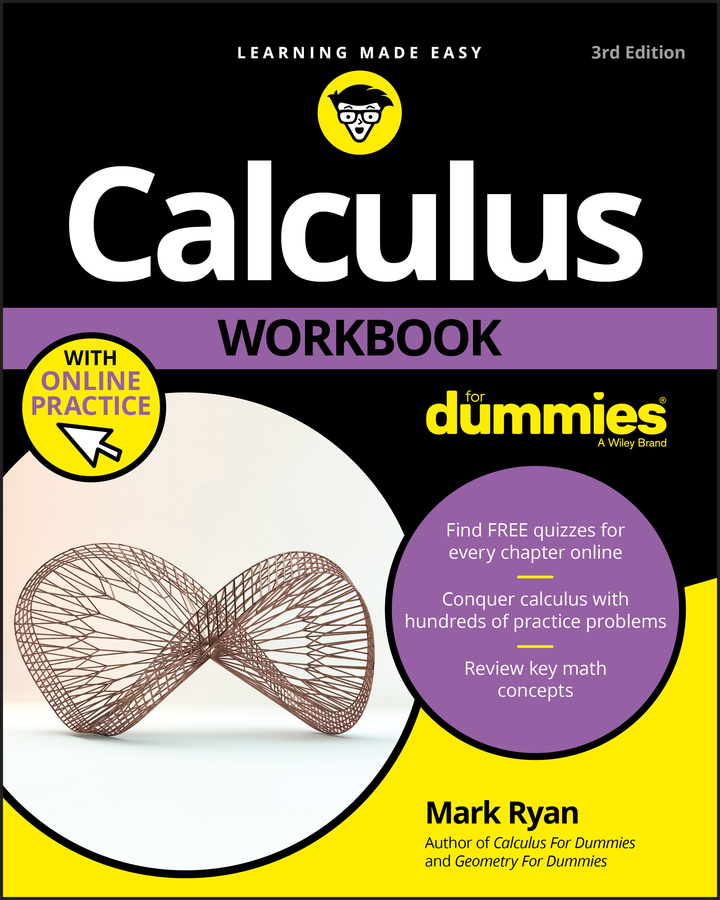Solving for limits at infinity is easy to do when you use a calculator. For example, enter the below function in your calculator's graphing mode: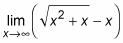then go to table setup and set TblStart to 100,000 and ∆Tbl to 100,000.

The table below shows the results.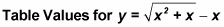X y
100,000 .4999988
200,000 .4999994
300,000 .4999996
400,000 .4999997
500,000 .4999998
600,000 .4999998
700,000 .4999998
800,000 .4999998
900,000 .4999999

You can see that y is getting extremely close to 0.5 as x gets larger and larger. So, 0.5 is the limit of the function as x approaches positive infinity, and there’s a horizontal asymptote at y = 0.5.

If you have any doubt that the limit equals 0.5, go back to table setup and put in a humongous TblStart and ∆Tbl, say 1,000,000,000, and check the table results again. All you see is a column of 0.5s. That’s the limit.

(By the way, the limit of this function as x approaches negative infinity doesn’t equal the limit as x approaches positive infinity: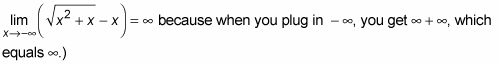One more thing: Just as with regular limits, using a calculator for infinite limits won’t give you an exact answer unless the numbers in the table are getting close to a number you recognize, like 0.5. If, for example, the exact answer to the limit is something like 5/17, which equals 0.2941…, when you see values in the table getting close to 0.2941, you probably won’t know that the values are approaching 5/17. You will, however, at least have an approximate answer to the limit problem.

Substitution does not work for the problem you solved above,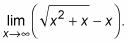If you plug ∞ into x, you get ∞ – ∞, which does not equal zero. A result of ∞ – ∞ tells you nothing about the answer to a limit problem.